1. 网站首页
2. 汽车精品
3. 产品详情

[A] ARB
[A] AEV
[A] AVM
[A] ATX
[A] ACRE
[A] ATL
[A] AMP
[A] AIR LIFT
[A] AMI
[A] ANZO
[A] AP
[A] 阿尔派
[B] Bushwacker
[B] BF Goodrich
[B] BANKS
[B] BILSTEIN
[B] Bestop
[B] Borla
[B] brembo
[B] BRP
[C] chrome
[C] CROWN
[C] COGNITO
[C] cargo-ease
[C] Crzay Cart
[C] Cleveland
[D] Dobinsons
[D] DAYSTAR
[D] DBA
[D] DANA
[D] DELTA

S/B-JEEP牧马人18款+ 备胎加宽支座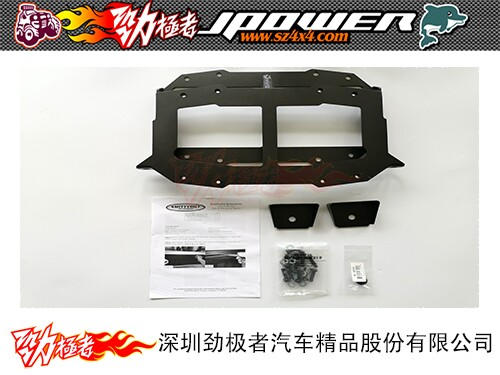3/16"激光切割钢I重11磅。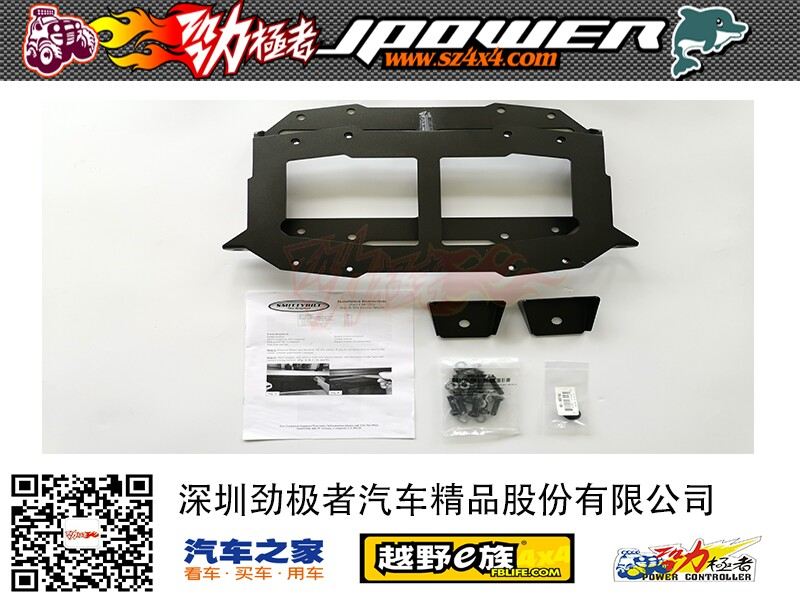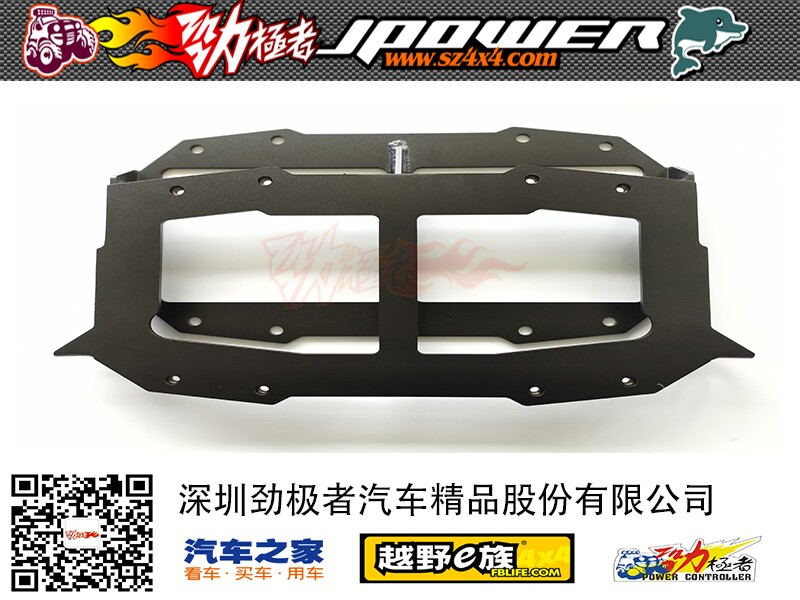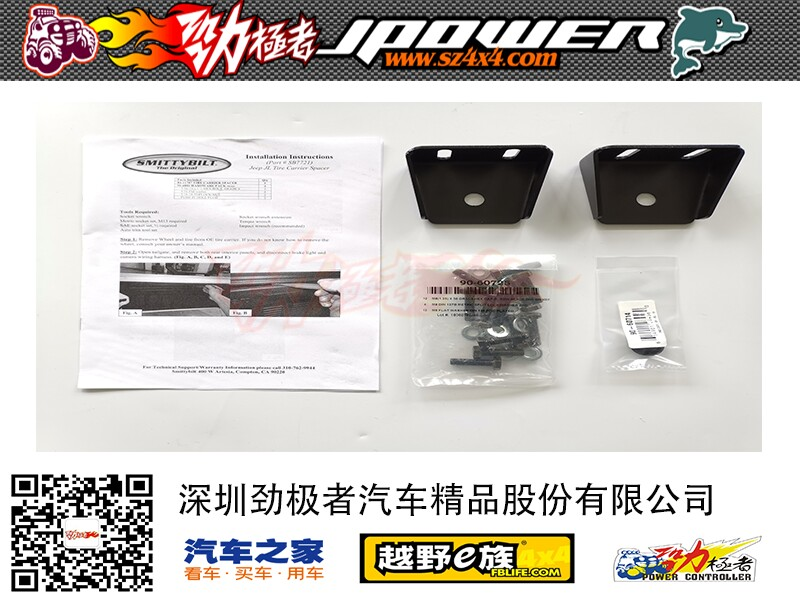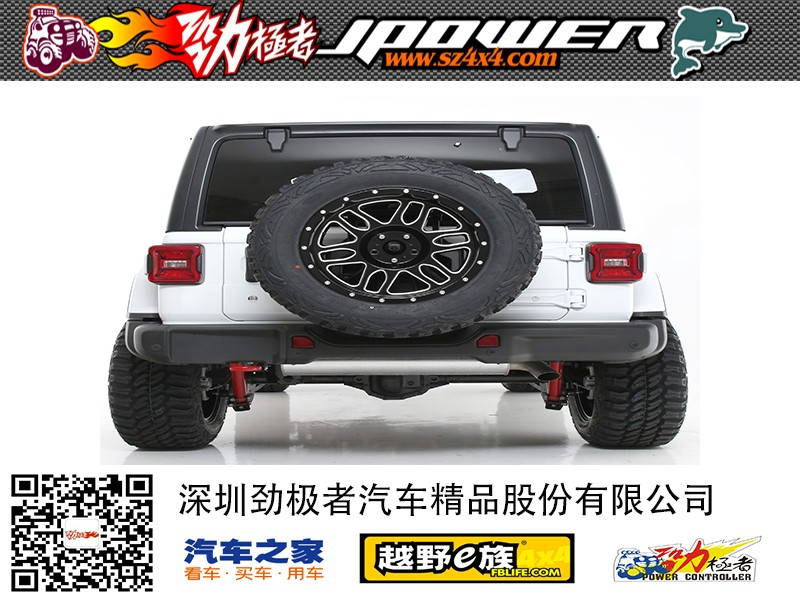#### 卢先生固定电话:0755-82948985 QQ:2355703632 电话：13530000737[A] ARB
[A] AEV
[A] AVM
[A] ATX
[A] ACRE
[A] ATL
[A] AMP
[A] AIR LIFT
[A] AMI
[A] ANZO
[A] AP
[A] 阿尔派
[B] Bushwacker
[B] BF Goodrich
[B] BANKS
[B] BILSTEIN
[B] Bestop
[B] Borla
[B] brembo
[B] BRP
[C] chrome
[C] CROWN
[C] COGNITO
[C] cargo-ease
[C] Crzay Cart
[C] Cleveland
[D] Dobinsons
[D] DAYSTAR
[D] DBA
[D] DANA
[D] DELTA
[D] Diablosport
[D] Diak CEPEK
[E] EBC
[E] ENGEL
[E] EVO
[F] FOX
[F] FAB
[F] Fabtech
[G] 国产
[G] GPS
[G] GIBSON
[G] G2
[G] GIBBS
[G] GoPro Hero
[H] Hella
[H] HI-LIFT
[H] hiddenhitch
[H] HONDA
[H] 环松
[I] IPF
[I] IRONMAN
[I] INDEL B
[I] ICI
[J] JET
[J] Jaos
[J] JOHNLITE
[J] J.POWER
[J] JEEP
[J] Jet Boards
[J] J.W. Speaker
[J] JetSurf
[K] KING
[K] KN
[K] KC
[K] KOALA
[K] K-MAN
[L] lightforce
[L] LONG RANGER
[L] 赖仕雷恩
[L] Lily
[M] MileMarker
[M] MONTAGUE
[M] MOPAR
[M] MOTOMETAL
[M] Magnuson
[M] mytop
[N] NITTO
[O] OME
[O] Optima
[O] OUTBACK
[O] OMP
[O] ORT
[P] Pro-comp
[P] PREDATOR
[P] Power controller
[P] PERMA-COOL
[P] POWER SLOT
[P] POSI QUIET
[P] 庞巴迪 ATV
[P] PE
[P] PROCHARGER
[P] PIAA
[P] POISON SPYDER
[P] PRIDE
[Q] 其它
[R] Rancho
[R] RDA
[R] REEL
[R] RBP
[R] Rigid Industries
[R] RAMPAGE
[R] RUBICON
[R] Rugged Ridge
[R] RIPP
[R] RETRAX
[R] RAPTOR
[R] RCV
[R] Razor
[S] Skyjacker
[S] Steelhorse
[S] SmittyBilt
[S] sunnyland
[S] Standard Gear
[S] SZ
[S] SCEPTER
[S] SUPER SWAMPER
[S] SANJ
[S] SAFARI
[S] SPARCO
[S] StarCraft
[S] STAR
[S] Super Pro
[S] SNUGTOP
[S] SNAIL
[S] Suree
[T] TJM
[T] TRD
[T] 台湾
[T] TOUGH DOG
[T] T-MAX
[T] THULE
[T] Total Chaos
[T] TFI
[T] Teraflex
[T] Truck Lite
[T] TMD
[U] Unichip
[V] VALUE GEAR
[V] virtuix omni
[W] Warn
[W] Wild Boar
[W] WAECO唯固
[W] WeatherTeach
[X] XENON
[X] XD